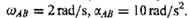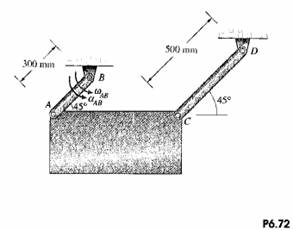### Create an Account

Already have account?

### Forgot Your Password ?

Home / Questions / The angular velocity and angular acceleration of bar AB are The dimensions of the rectang...

# The angular velocity and angular acceleration of bar AB are The dimensions of the rectangular plate are 300 mm 600 mm What are the angular velocity and angular acceleration of the rectangular

The angular velocity and angular acceleration of bar AB areThe dimensions of the rectangular plate are 300 mm x 600 mm, What are the angular velocity and angular acceleration of the rectangular plate?Jun 16 2020 View more View Less

#### Answer (Solved)Subscribe To Get Solution